# Basic Astronomy

Source: Open Course Library

Student Price: FREE

A collection of Astronomy quizzes from Wright State University.

# Basic Astronomy I

Q1

The range of wavelength for visible light is between

A

600 and 1200 nanometers

B

400 and 700 nanometers

C

0.1 and 10 nanometers

D

5000 and 6000 nanometers

E

1 and 10 nanometers

Q2

The number of globular clusters in the Milky way galaxy is about

A

15 million

B

150

C

15 thousand

D

1,500

Q3

$KE={\frac{4\pi^{2}}{5}}\frac{MR^{2}}{P^{2}}$ is the kinetic energy of a solid rotating ball, where M is mass, R is radius, and P is period. And, $Power=\frac{energy}{time}$. You are banging espressos in a little coffeehouse with your astronomy friends, talking about a new SN remnant that closely resembles the Crab. You have observed the pulsar, and wonder what the total power output of the nebula might be. You know both the period of the pulsar, as well as , which represents the amount of time you think the pulsar will continue pulsing if it continues slowing down at its present rate. What formula do you write on your napkin?

A

$Power={\frac{4T\pi^{2}}{5}}\frac{MR^{2}}{P^{2}}$

B

$Power={\frac{4\pi^{2}}{5T^{2}}}\frac{MR^{2}}{P^{2}}$

C

$Power={\frac{5}{4T\pi^{2}}}\frac{MR^{2}}{P^{2}}$

D

$Power={\frac{4\pi^{2}}{5T}}\frac{MR^{2}}{P^{2}}$

E

$Power={\frac{4\pi^{2}}{5}}\frac{MR^{2}}{P^{2}}T^4$

Q4

The Hayashi and Henyey tracks refer to how T Tauri of different masses will move

A

through an HR diagram as they are born

B

Two of these are true

C

through a cluster as they are born

D

through a cluster as they die

E

through an HR diagram as they die

Q5

A dying star with more than 1.4 solar masses becomes a ______________, and those with more than 5 solar masses becomes a ______________

A

white dwarf....neutron star

B

white dwarf...red dwarf

C

white dwarf....black hole

D

neutron star....black hole

E

blue giant....red giant

Q6

Place yourself in an expanding raisinbread model of Hubble expansion. A raisin originally situated at a distance of 2 cm expands out to 4 cm. To what distance would a raisin originally situated at a distance of 4 cm expand?

A

4

B

3

C

2

D

6

E

8

Q7

What causes the blue glow of the Crab nebula?

A

the curving motion of electrons in a magnetic field; such motion resembles a radio antenna

B

the same emission found in a Lava lamp (ultraviolet)

C

the Gravitational blue shift

D

the curving motion of electrons in a magnetic field; such motion traps ultraviolet and blue light

E

the Doppler blue shift

Q8

Many stars in a typical open cluster are nearly as old as the universe

A

True

B

False

Q9

Stars that begin with more than 50 solar masses will typically lose ______________ while on the main sequence.

A

10% of their magnetic field

B

10% their mass

C

50% their mass

D

1% their mass

E

all of their magnetic field

Q10

You at the center raisin of an expanding raisinbread model of Hubble expansion, and from your location a raisin originally situated at a distance of 1 cm expands out to a distance of 4 cm. The nearest raisin with intelligent life is situated exactly halfway between your (central) location and the edge. How would this second "intelligent" raisin view an expansion of a raisin 1 cm away?

A

expansion from 1 cm to 9 cm (since 51= 4)

B

expansion from 1 cm to 4 cm (just like yours).

C

expansion from 1 cm to 8 cm (twice yours).

D

expansion from 1 cm to 2 cm (half of yours)

E

expansion from 1 cm to 3 cm (since 31= 2)

Q11

Stellar parallax is

A

an annual change in angular position of a star as seen from Earth

B

an astronomical object with known luminosity.

C

a numerical measure of brightness as seen from Earth

D

a numerical measure of brightness as seen from a distance of approximately 33 lightyears

E

the total amount of energy emitted per unit time.

Q12

Members of an open cluster feel significant forces only due to gravitational interaction with each other

A

True

B

False

Q13

Pre–main sequence stars are often surrounded by a protoplanetary disk and powered mainly by

A

chemical reactions

B

the fusion of Helium to Carbon

C

collisions between protoplanets

D

the fission of Carbon from Helium

E

the release of gravitational energy

Q14

What was Messier doing when he independently rediscovered the Crab in 1758?

A

Attempting one of the first star charts

B

Looking for a comet that he knew would be appearing in that part of the sky.

C

Attempting to count asteroids

D

Trying to measure the orbital radius of a planet

E

Looking for lobsters

Q15

In 1989 the satellite Hipparcos was launched primarily for obtaining parallaxes and proper motions allowing measurements of stellar parallax for stars up to about 500 parsecs away, which is about ______________ times the diameter of the Milky Way Galaxy.

A

15

B

1.5

C

.015

D

0.15

E

150

Q16

Members of a globular cluster tend to be

A

old

B

of all ages

C

young

Q17

What causes the "fingerlike" filamentary structure in the Crab nebula?

A

a heavy (high density) fluid underneath a light (low density) fluid, like a lava lamp

B

electrons striking oxygen molecules, like a lava lamp

C

electrons striking hydrogen molecules, like a lava lamp

D

a light(low density) fluid underneath a heavy(high density) fluid, like a lava lamp

E

cyclotron motion, causing the electrons to strike oxygen molecules

Q18

In one respect, the universie is arguably "young", considering how much complexity it contains. This is often illustrated by a calculation of

A

cosmic expansion

B

recalibration of supernovae relative magnitude

C

cosmic redshift

D

chimps typing Shakespeare

E

recalibration of supernovae luminosity

Q19

When a star with more than 10 solar masses ceases fuse hydrogen to helium, it

A

it fuses helium to carbon to iron (and other elements), then continues to release more energy by fusing the iron to heavier elements such as uranium.

B

ceases to convert nuclear energy.

C

it fuses helium to carbon and other elements up to iron and then ceases to produce more energy

D

it fuses helium to carbon and then ceases to produce more energy

E

it fuses elements up to uranium, and continues to produce energy by the fission of uranium.

Q20

Based on the HR diagrams and images in stars shown in the materials, a very large red supergiant has a diameter that is about ______________ greater than a small white dwarf.

A

3x10$^{11}$

B

3x10$^7$

C

3x10$^9$

D

3x10$^5$

E

3x10$^3$

Q21

The location of open clusters can be described as

A

uniformly distributed within the galactic disk

B

in the spiral arms

C

between the spiral arms

D

uniformly distributed in a sphere centered at the Milky Way's center

Q22

According to Wikipedia, a star with over 20 solar masses converts its Hyrogen to Helium in about 8 billion years, but the conversion of Oxygen to heavier elements take about ______________

A

1 thousand years

B

1 million years

C

1 billion years

D

10 billion years

E

1 year

Q23

An object emits thermal (blackbody) radiation with a peak wavelength of 250nm. How does its temperature compare with the Sun?

A

2 times hotter than the Sun

B

5 times colder than the Sun

C

The temperature is the same

D

2 times colder than the Sun

E

5 times hotter than the Sun

Q24

Most globular clusters that we see in the sky orbit ______________ and have ______________ orbits

A

the center of the Milky way ... nearly circular

B

within the disk of the Milky way ... nearly circular

C

within the disk of the Milky way ... elliptic orbits

D

the center of the Milky way ... elliptic orbits

Q25

Members of a globular cluster tend to have

A

low mass

B

high mass

C

a wide range of masses

Q26

Luminosity is

A

a numerical measure of brightness as seen from a distance of approximately 33 lightyears

B

an astronomical object with known luminosity.

C

an annual change in angular position of a star as seen from Earth

D

a numerical measure of brightness as seen from Earth

E

the total amount of energy emitted per unit time.

Q27

What happens if you increase the size of a giant molecular cloud while keeping temperature and mass fixed?

A

It is more likely to collapse because this will increase the temperature

B

It is more likely to collapse because larger things have more gravity

C

It is less likely to collapse because temperature can never be kept fixed

D

It is less likely to collapse spreading it out weakens the force of gravity

E

It is equally likely to collapse because size is not part of the Jean's criterion.

Q28

The course materials presented three arguments suggesting that a white dwarf is roughly the size of the earth. Which best summarizes them?

A

x-ray-emmission... doppler-shift... rotation-rate

B

HR-diagram-location... X-ray-emmision... spectral-lines

C

all of these are true

D

temperature-luminosity... redshift...quantum-theory-of-solids

E

doppler-shift... period-of-pulsation... temperature-luminosity

Q29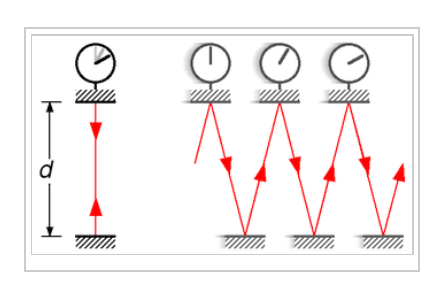This light clock is associated with

A

general relativity

B

special relativity

C

all of these are true

D

gravitational shift

E

doppler shift

Q30

Place yourself in an expanding raisinbread model of Hubble expansion. A raisin originally situated at a distance of 4 cm expands out to 12 cm. To what distance would a raisin originally situated at a distance of 2 cm expand?

A

6

B

2

C

4

D

8

E

3

Q31

Members of an open cluster feel significant forces from nearby giant molecular clouds

A

True

B

False

Q32

Absolute magnitude is

A

an annual change in angular position of a star as seen from Earth

B

the total amount of energy emitted per unit time.

C

a numerical measure of brightness as seen from a distance of approximately 33 lightyears

D

an astronomical object with known luminosity.

E

a numerical measure of brightness as seen from Earth

Q33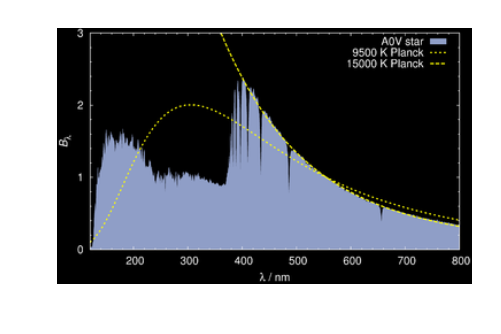This spectrum of the star Vega suggests that

A

if is not really a black body

B

all of these are true

C

it's surface can be associated with a range of temperatures

D

it can be associated with an "effective" temperature

E

it is an approximate black body

Q34

A starburst galaxy.

A

usually is a result of collisions between galaxies

B

All of these are correct

C

Two of these are correct

D

is a region of active stellar birth

E

has only dead or dying stars

Q35

One way to determine the distance to a nebula or small cluster of clouds is to compare the angular expansion to the spectroscopic Doppler shift. Two clusters (A and B) have the same spectroscopically measured velocity. Cluster A is moving towards the observer and exhibits the greater angular expansion. Which cluster is closer?

A

cluster A, because it exhibits greater angular expansion

B

either cluster might be more distant

C

cluster A, because it exhibits a blue Doppler shift

D

cluster B, because it exhibits a red Doppler shift

E

cluster B, because it exhibits less angular expansion

# Basic Astronomy II

Q1

Place yourself in an expanding raisinbread model of Hubble expansion. A raisin originally situated at a distance of 2 cm expands out to 4 cm. To what distance would a raisin originally situated at a distance of 4 cm expand?

A

2

B

4

C

6

D

8

E

3

Q2

Based on the HR diagrams and images in stars shown in the materials, a very large red supergiant has a diameter that is about ______________ greater than a small white dwarf.

A

3x10$^7$

B

3x10$^3$

C

3x10$^9$

D

3x10$^5$

E

3x10$^{11}$

Q3

Members of a globular cluster tend to be

A

young

B

of all ages

C

old

Q4

A starburst galaxy.

A

usually is a result of collisions between galaxies

B

Two of these are correct

C

has only dead or dying stars

D

is a region of active stellar birth

E

All of these are correct

Q5

The range of wavelength for visible light is between

A

400 and 700 nanometers

B

0.1 and 10 nanometers

C

600 and 1200 nanometers

D

5000 and 6000 nanometers

E

1 and 10 nanometers

Q6

In one respect, the universie is arguably "young", considering how much complexity it contains. This is often illustrated by a calculation of

A

recalibration of supernovae relative magnitude

B

recalibration of supernovae luminosity

C

chimps typing Shakespeare

D

cosmic expansion

E

cosmic redshift

Q7

One way to determine the distance to a nebula or small cluster of clouds is to compare the angular expansion to the spectroscopic Doppler shift. Two clusters (A and B) have the same spectroscopically measured velocity. Cluster A is moving towards the observer and exhibits the greater angular expansion. Which cluster is closer?

A

cluster A, because it exhibits greater angular expansion

B

cluster B, because it exhibits a red Doppler shift

C

cluster A, because it exhibits a blue Doppler shift

D

either cluster might be more distant

E

cluster B, because it exhibits less angular expansion

Q8

A dying star with more than 1.4 solar masses becomes a ______________, and those with more than 5 solar masses becomes a ______________

A

white dwarf....black hole

B

neutron star....black hole

C

white dwarf....neutron star

D

blue giant....red giant

E

white dwarf...red dwarf

Q9

Members of a globular cluster tend to have

A

low mass

B

high mass

C

a wide range of masses

Q10

When a star with more than 10 solar masses ceases fuse hydrogen to helium, it

A

it fuses elements up to uranium, and continues to produce energy by the fission of uranium.

B

ceases to convert nuclear energy.

C

it fuses helium to carbon and then ceases to produce more energy

D

it fuses helium to carbon to iron (and other elements), then continues to release more energy by fusing the iron to heavier elements such as uranium.

E

it fuses helium to carbon and other elements up to iron and then ceases to produce more energy

Q11

Many stars in a typical open cluster are nearly as old as the universe

A

True

B

False

Q12

An object emits thermal (blackbody) radiation with a peak wavelength of 250nm. How does its temperature compare with the Sun?

A

5 times hotter than the Sun

B

The temperature is the same

C

5 times colder than the Sun

D

2 times colder than the Sun

E

2 times hotter than the Sun

Q13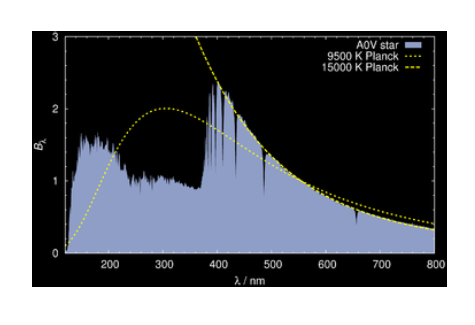This spectrum of the star Vega suggests that

A

if is not really a black body

B

it can be associated with an "effective" temperature

C

it is an approximate black body

D

all of these are true

E

it's surface can be associated with a range of temperatures

Q14

Pre–main sequence stars are often surrounded by a protoplanetary disk and powered mainly by

A

the release of gravitational energy

B

the fusion of Helium to Carbon

C

chemical reactions

D

collisions between protoplanets

E

the fission of Carbon from Helium

Q15

Absolute magnitude is

A

an astronomical object with known luminosity.

B

a numerical measure of brightness as seen from Earth

C

a numerical measure of brightness as seen from a distance of approximately 33 lightyears

D

the total amount of energy emitted per unit time.

E

an annual change in angular position of a star as seen from Earth

Q16

Most globular clusters that we see in the sky orbit ______________ and have ______________ orbits

A

within the disk of the Milky way ... elliptic orbits

B

within the disk of the Milky way ... nearly circular

C

the center of the Milky way ... elliptic orbits

D

the center of the Milky way ... nearly circular

Q17

The Hayashi and Henyey tracks refer to how T Tauri of different masses will move

A

through a cluster as they are born

B

through an HR diagram as they are born

C

Two of these are true

D

through a cluster as they die

E

through an HR diagram as they die

Q18

You at the center raisin of an expanding raisinbread model of Hubble expansion, and from your location a raisin originally situated at a distance of 1 cm expands out to a distance of 4 cm. The nearest raisin with intelligent life is situated exactly halfway between your (central) location and the edge. How would this second "intelligent" raisin view an expansion of a raisin 1 cm away?

A

expansion from 1 cm to 3 cm (since 31= 2)

B

expansion from 1 cm to 4 cm (just like yours).

C

expansion from 1 cm to 9 cm (since 51= 4)

D

expansion from 1 cm to 8 cm (twice yours).

E

expansion from 1 cm to 2 cm (half of yours)

Q19

The number of globular clusters in the Milky way galaxy is about

A

1,500

B

15 thousand

C

15 million

D

150

Q20

Place yourself in an expanding raisinbread model of Hubble expansion. A raisin originally situated at a distance of 4 cm expands out to 12 cm. To what distance would a raisin originally situated at a distance of 2 cm expand?

A

3

B

6

C

2

D

4

E

8

Q21

What causes the blue glow of the Crab nebula?

A

the same emission found in a Lava lamp (ultraviolet)

B

the Doppler blue shift

C

the curving motion of electrons in a magnetic field; such motion resembles a radio antenna

D

the curving motion of electrons in a magnetic field; such motion traps ultraviolet and blue light

E

the Gravitational blue shift

Q22

In 1989 the satellite Hipparcos was launched primarily for obtaining parallaxes and proper motions allowing measurements of stellar parallax for stars up to about 500 parsecs away, which is about ______________ times the diameter of the Milky Way Galaxy.

A

.015

B

1.5

C

15

D

150

E

0.15

Q23

What happens if you increase the size of a giant molecular cloud while keeping temperature and mass fixed?

A

It is more likely to collapse because larger things have more gravity

B

It is less likely to collapse because temperature can never be kept fixed

C

It is equally likely to collapse because size is not part of the Jean's criterion.

D

It is more likely to collapse because this will increase the temperature

E

It is less likely to collapse spreading it out weakens the force of gravity

Q24

Members of an open cluster feel significant forces from nearby giant molecular clouds

A

True

B

False

Q25

What causes the "fingerlike" filamentary structure in the Crab nebula?

A

electrons striking oxygen molecules, like a lava lamp

B

cyclotron motion, causing the electrons to strike oxygen molecules

C

electrons striking hydrogen molecules, like a lava lamp

D

a heavy (high density) fluid underneath a light (low density) fluid, like a lava lamp

E

a light(low density) fluid underneath a heavy(high density) fluid, like a lava lamp

Q26

According to Wikipedia, a star with over 20 solar masses converts its Hyrogen to Helium in about 8 billion years, but the conversion of Oxygen to heavier elements take about ______________

A

10 billion years

B

1 million years

C

1 billion years

D

1 thousand years

E

1 year

Q27

Stellar parallax is

A

the total amount of energy emitted per unit time.

B

an annual change in angular position of a star as seen from Earth

C

a numerical measure of brightness as seen from Earth

D

a numerical measure of brightness as seen from a distance of approximately 33 lightyears

E

an astronomical object with known luminosity.

Q28

The location of open clusters can be described as

A

in the spiral arms

B

between the spiral arms

C

uniformly distributed within the galactic disk

D

uniformly distributed in a sphere centered at the Milky Way's center

Q29

Members of an open cluster feel significant forces only due to gravitational interaction with each other

A

True

B

False

Q30

Luminosity is

A

a numerical measure of brightness as seen from a distance of approximately 33 lightyears

B

an annual change in angular position of a star as seen from Earth

C

the total amount of energy emitted per unit time.

D

an astronomical object with known luminosity.

E

a numerical measure of brightness as seen from Earth

Q31

Stars that begin with more than 50 solar masses will typically lose ______________ while on the main sequence.

A

10% of their magnetic field

B

10% their mass

C

all of their magnetic field

D

1% their mass

E

50% their mass

Q32

What was Messier doing when he independently rediscovered the Crab in 1758?

A

Attempting one of the first star charts

B

Looking for a comet that he knew would be appearing in that part of the sky.

C

Attempting to count asteroids

D

Trying to measure the orbital radius of a planet

E

Looking for lobsters

Q33

The course materials presented three arguments suggesting that a white dwarf is roughly the size of the earth. Which best summarizes them?

A

x-ray-emmission... doppler-shift... rotation-rate

B

HR-diagram-location... X-ray-emmision... spectral-lines

C

temperature-luminosity... redshift...quantum-theory-of-solids

D

doppler-shift... period-of-pulsation... temperature-luminosity

E

all of these are true

Q34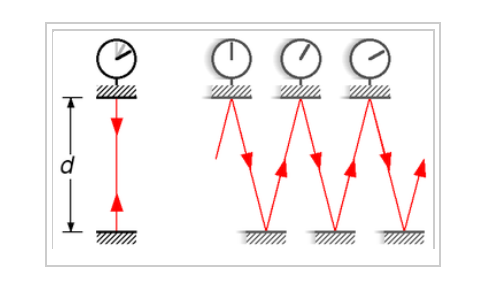This light clock is associated with

A

doppler shift

B

general relativity

C

all of these are true

D

gravitational shift

E

special relativity

Q35

$KE={\frac{4\pi^{2}}{5}}\frac{MR^{2}}{P^{2}}$ is the kinetic energy of a solid rotating ball, where M is mass, R is radius, and P is period. And, $Power=\frac{energy}{time}$. You are banging espressos in a little coffeehouse with your astronomy friends, talking about a new SN remnant that closely resembles the Crab. You have observed the pulsar, and wonder what the total power output of the nebula might be. You know both the period of the pulsar, as well as , which represents the amount of time you think the pulsar will continue pulsing if it continues slowing down at its present rate. What formula do you write on your napkin?

A

$Power={\frac{4\pi^{2}}{5}}\frac{MR^{2}}{P^{2}}T^4$

B

$Power={\frac{4T\pi^{2}}{5}}\frac{MR^{2}}{P^{2}}$

C

$Power={\frac{5}{4T\pi^{2}}}\frac{MR^{2}}{P^{2}}$

D

$Power={\frac{4\pi^{2}}{5T}}\frac{MR^{2}}{P^{2}}$

E

$Power={\frac{4\pi^{2}}{5T^{2}}}\frac{MR^{2}}{P^{2}}$

# Basic Astronomy III

Q1

Many stars in a typical open cluster are nearly as old as the universe

A

True

B

False

Q2

A grouping with a hundred stars is probably a

A

elliptical galaxy

B

open cluster

C

globular cluster

D

dwarf galaxy

E

AB association

Q3

Giant molecular clouds with sufficient conditions to form a star cluster would have formed them long ago. Any stellar births in the past couple of billions years probably resulted from ______________ between clouds.

A

None of these is correct.

B

collisions

C

ion exchange

D

Two of these are correct

E

photon exchange

Q4

A dying star with more than 1.4 solar masses becomes a ______________, and those with more than 5 solar masses becomes a ______________

A

neutron star....black hole

B

blue giant....red giant

C

white dwarf....neutron star

D

white dwarf....black hole

E

white dwarf...red dwarf

Q5

Why is a star made of plasma?

A

plasma is generic word for "important"

B

plasma is always present when there are strong magnetic fields

C

it is so hot that electrons are stripped away from the protons

D

the interstellar gas was mostly plasma

E

the intense gravity liquifies the substance, just as red blood cells liquify plasma in the body

Q6

Place yourself in an expanding raisinbread model of Hubble expansion. A raisin originally situated at a distance of 2 cm expands out to 4 cm. To what distance would a raisin originally situated at a distance of 4 cm expand?

A

8

B

3

C

2

D

4

E

6

Q7

Members of an open cluster feel significant forces from nearby giant molecular clouds

A

True

B

False

Q8

What causes the blue glow of the Crab nebula?

A

the Gravitational blue shift

B

the Doppler blue shift

C

the same emission found in a Lava lamp (ultraviolet)

D

the curving motion of electrons in a magnetic field; such motion traps ultraviolet and blue light

E

the curving motion of electrons in a magnetic field; such motion resembles a radio antenna

Q9

The range of wavelength for visible light is between

A

1 and 10 nanometers

B

400 and 700 nanometers

C

5000 and 6000 nanometers

D

0.1 and 10 nanometers

E

600 and 1200 nanometers

Q10

What is the difference between a constellation and an asterism?

A

constellations represent regions of the sky, like state boundaries on a map of the USA

B

asterisms are larger than constellations

C

constellations consist of never more than ten stars.

D

none of these is correct

E

asterisms are smaller than constellations

Q11

Based on the HR diagrams and images in stars shown in the materials, a very large red supergiant has a diameter that is about ______________ greater than a small white dwarf.

A

3x10$^{11}$

B

3x10$^5$

C

3x10$^7$

D

3x10$^9$

E

3x10$^3$

Q12

The "normalized intensity" of a Sunlike star situated one parsec from Earth would be 4πI = 1. What is 4πI for a star with 100 times the Sun's energy output that is situated 10pc from Earth?

A

10$^{-2}$

B

10$^{-1}$

C

10$^{-4}$

D

1

E

10$^{-3}$

Q13

Which of the following changes in the properties of a giant molecular cloud might cause it to collapse?

A

Increase size at fixed pressure and mass

B

Two of these are correct

C

Increase temperature at fixed mass and size

D

Increase mass at fixed temperature and size

E

Decrease mass at fixed temperature and size

Q14

Most globular clusters that we see in the sky orbit ______________ and have ______________ orbits

A

the center of the Milky way ... elliptic orbits

B

within the disk of the Milky way ... nearly circular

C

the center of the Milky way ... nearly circular

D

within the disk of the Milky way ... elliptic orbits

Q15

Relative magnitude is

A

an astronomical object with known luminosity.

B

an annual change in angular position of a star as seen from Earth

C

the total amount of energy emitted per unit time.

D

a numerical measure of brightness as seen from Earth

E

a numerical measure of brightness as seen from a distance of approximately 33 lightyears

Q16

What happens if you increase the size of a giant molecular cloud while keeping temperature and mass fixed?

A

It is more likely to collapse because larger things have more gravity

B

It is less likely to collapse because temperature can never be kept fixed

C

It is equally likely to collapse because size is not part of the Jean's criterion.

D

It is more likely to collapse because this will increase the temperature

E

It is less likely to collapse spreading it out weakens the force of gravity

Q17

Members of an open cluster feel significant forces only due to gravitational interaction with each other

A

True

B

False

Q18

Comparing Hubble's original (1929) plot of redshift versus distance with the later one in 2007, the latter extends farther into space by a factor of

A

100

B

1000

C

10,000

D

100,000

E

10

Q19

The course materials present two cosmic expansion plots. The more recent (2007) plot used

A

novae

B

entire galaxies

C

supernovae

D

Cepheid variables

E

red giants

Q20

A grouping with 100 thousand stars would probably be a

A

globular cluster

B

AB association

C

dwarf galaxy

D

elliptical galaxy

E

open cluster

Q21

A standard candle is

A

an annual change in angular position of a star as seen from Earth

B

a numerical measure of brightness as seen from Earth

C

a numerical measure of brightness as seen from a distance of approximately 33 lightyears

D

the total amount of energy emitted per unit time.

E

an astronomical object with known luminosity.

Q22

Place yourself in an expanding raisinbread model of Hubble expansion. A raisin originally situated at a distance of 4 cm expands out to 12 cm. To what distance would a raisin originally situated at a distance of 2 cm expand?

A

2

B

4

C

6

D

3

E

8

Q23

A star that is increasing it's temperature while maintaining constant luminosity is

A

getting smaller in size

B

on the verge of becoming a supernovae

C

in the process of dying

D

turning red

E

getting larger in size

Q24

An object emits thermal (blackbody) radiation with a peak wavelength of 250nm. How does its temperature compare with the Sun?

A

5 times colder than the Sun

B

2 times colder than the Sun

C

The temperature is the same

D

2 times hotter than the Sun

E

5 times hotter than the Sun

Q25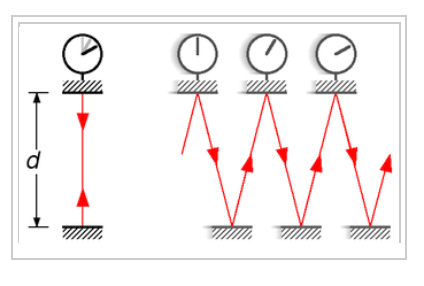Suppose the light clock involved a ball being tossed back and forth on a train going just under the speed of sound. In contrast to the situation for light reflecting back and forth on a train going just under the speed of light, there is virtually no time dilation. Why?

A

Special relativity is valid only for objects travelling in a vacuum.

B

The observer on the ground would perceive the ball to be travelling faster.

C

The observer on the ground would perceive the width the train to be smaller.

D

The observer on the ground would perceive the width the train to be greater.

E

The observer on the ground would perceive the ball to be travelling more slowly.

Q26

Many supernovae begin as a shock wave in the core that was caused by

A

Many supernovae begin as a shock wave in the core that was caused by

B

carbon and other elements fusing into iron

C

iron fusing into heavier elements such as uranium

D

the conversion of carbon into diamonds,

E

electrons being driven into protons to form neutrons

Q27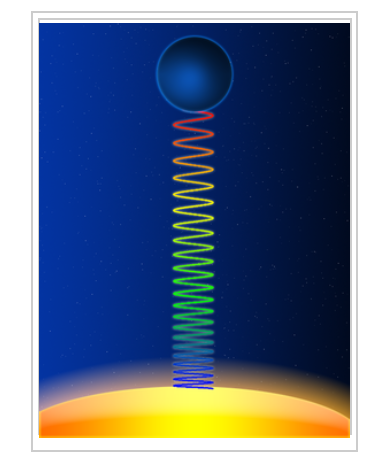What best explains this figure?

A

The photon slows down, by the Doppler shift, E=hf, and therefore by c=f&;lambda it turns red.

B

The photon slows down, by the Doppler shift, c=fλ, and therefore by E=hf it turns red.

C

The photon loses energy, not speed. By E=hf, it loses frequency, and by c=fλ it increases wavelength and turns red.

D

The photon loses energy, not speed. By c=fλ , it loses frequency, and by E=hf it increases wavelength and turns red.

E

The photon slows down as it goes uphill, and by c=fλ it increases wavelength therefore by E=hf, it turns red.

Q28

What was Messier doing when he independently rediscovered the Crab in 1758?

A

Looking for lobsters

B

Trying to measure the orbital radius of a planet

C

Looking for a comet that he knew would be appearing in that part of the sky.

D

Attempting one of the first star charts

E

Attempting to count asteroids

Q29

The course materials present two cosmic expansion plots. Hubble's original (1929) plot used

A

novae

B

entire galaxies

C

supernovae

D

Cepheid variables

E

red giants

Q30

Members of a globular cluster tend to have

A

a wide range of masses

B

low mass

C

high mass

Q31

Many stars in a typical globular cluster are nearly as old as the universe

A

True

B

False

Q32

Aside from its location on the HR diagram, evidence that the white dwarf has a small radius can be found from

A

the doppler shift

B

the expansion of the universe

C

the temperature

D

the mass as measured by Kepler's third law (modified by Newton)

E

the gravitational redshift

Q33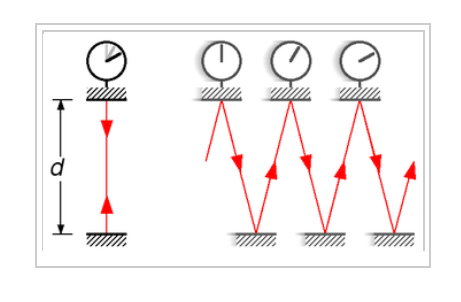This light clock is associated with

A

gravitational shift

B

general relativity

C

doppler shift

D

special relativity

E

all of these are true

Q34

What causes the "fingerlike" filamentary structure in the Crab nebula?

A

a light(low density) fluid underneath a heavy(high density) fluid, like a lava lamp

B

cyclotron motion, causing the electrons to strike oxygen molecules

C

electrons striking hydrogen molecules, like a lava lamp

D

a heavy (high density) fluid underneath a light (low density) fluid, like a lava lamp

E

electrons striking oxygen molecules, like a lava lamp

Q35

A starburst galaxy.

A

All of these are correct

B

usually is a result of collisions between galaxies

C

has only dead or dying stars

D

Two of these are correct

E

is a region of active stellar birth

# Basic Astronomy IV

Q1

Many stars in a typical open cluster are nearly as old as the universe

A

True

B

False

Q2

Members of a globular cluster tend to have

A

low mass

B

a wide range of masses

C

high mass

Q3

Relative magnitude is

A

an annual change in angular position of a star as seen from Earth

B

an astronomical object with known luminosity.

C

a numerical measure of brightness as seen from a distance of approximately 33 lightyears

D

a numerical measure of brightness as seen from Earth

E

the total amount of energy emitted per unit time.

Q4

What causes the "fingerlike" filamentary structure in the Crab nebula?

A

cyclotron motion, causing the electrons to strike oxygen molecules

B

electrons striking hydrogen molecules, like a lava lamp

C

a heavy (high density) fluid underneath a light (low density) fluid, like a lava lamp

D

electrons striking oxygen molecules, like a lava lamp

E

a light(low density) fluid underneath a heavy(high density) fluid, like a lava lamp

Q5

Place yourself in an expanding raisinbread model of Hubble expansion. A raisin originally situated at a distance of 4 cm expands out to 12 cm. To what distance would a raisin originally situated at a distance of 2 cm expand?

A

3

B

2

C

6

D

8

E

4

Q6

A starburst galaxy.

A

has only dead or dying stars

B

is a region of active stellar birth

C

usually is a result of collisions between galaxies

D

All of these are correct

E

Two of these are correct

Q7

An object emits thermal (blackbody) radiation with a peak wavelength of 250nm. How does its temperature compare with the Sun?

A

5 times hotter than the Sun

B

5 times colder than the Sun

C

2 times hotter than the Sun

D

2 times colder than the Sun

E

The temperature is the same

Q8

Aside from its location on the HR diagram, evidence that the white dwarf has a small radius can be found from

A

the expansion of the universe

B

the temperature

C

the mass as measured by Kepler's third law (modified by Newton)

D

the doppler shift

E

the gravitational redshift

Q9

Giant molecular clouds with sufficient conditions to form a star cluster would have formed them long ago. Any stellar births in the past couple of billions years probably resulted from ______________ between clouds.

A

collisions

B

None of these is correct.

C

photon exchange

D

Two of these are correct

E

ion exchange

Q10

Place yourself in an expanding raisinbread model of Hubble expansion. A raisin originally situated at a distance of 2 cm expands out to 4 cm. To what distance would a raisin originally situated at a distance of 4 cm expand?

A

6

B

8

C

3

D

2

E

4

Q11

The "normalized intensity" of a Sunlike star situated one parsec from Earth would be 4πI = 1. What is 4πI for a star with 100 times the Sun's energy output that is situated 10pc from Earth?

A

10$^{-4}$

B

10$^{-2}$

C

1

D

10$^{-3}$

E

10$^{-1}$

Q12

A grouping with a hundred stars is probably a

A

open cluster

B

elliptical galaxy

C

dwarf galaxy

D

globular cluster

E

AB association

Q13

A star that is increasing it's temperature while maintaining constant luminosity is

A

in the process of dying

B

getting smaller in size

C

on the verge of becoming a supernovae

D

getting larger in size

E

turning red

Q14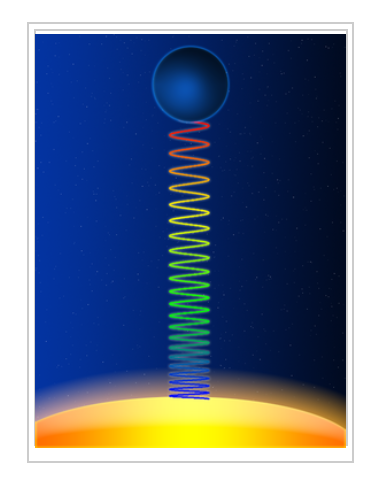What best explains this figure?

A

The photon slows down, by the Doppler shift, E=hf, and therefore by c=f&;lambda it turns red.

B

The photon slows down as it goes uphill, and by c=fλ it increases wavelength therefore by E=hf, it turns red.

C

The photon slows down, by the Doppler shift, c=fλ, and therefore by E=hf it turns red.

D

The photon loses energy, not speed. By E=hf, it loses frequency, and by c=fλ it increases wavelength and turns red.

E

The photon loses energy, not speed. By c=fλ , it loses frequency, and by E=hf it increases wavelength and turns red.

Q15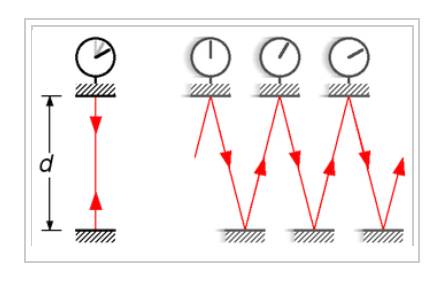Suppose the light clock involved a ball being tossed back and forth on a train going just under the speed of sound. In contrast to the situation for light reflecting back and forth on a train going just under the speed of light, there is virtually no time dilation. Why?

A

The observer on the ground would perceive the width the train to be smaller.

B

The observer on the ground would perceive the ball to be travelling faster.

C

The observer on the ground would perceive the ball to be travelling more slowly.

D

Special relativity is valid only for objects travelling in a vacuum.

E

The observer on the ground would perceive the width the train to be greater.

Q16

What is the difference between a constellation and an asterism?

A

constellations consist of never more than ten stars.

B

asterisms are smaller than constellations

C

constellations represent regions of the sky, like state boundaries on a map of the USA

D

asterisms are larger than constellations

E

none of these is correct

Q17

What happens if you increase the size of a giant molecular cloud while keeping temperature and mass fixed?

A

It is less likely to collapse spreading it out weakens the force of gravity

B

It is more likely to collapse because larger things have more gravity

C

It is less likely to collapse because temperature can never be kept fixed

D

It is equally likely to collapse because size is not part of the Jean's criterion.

E

It is more likely to collapse because this will increase the temperature

Q18

Members of an open cluster feel significant forces only due to gravitational interaction with each other

A

True

B

False

Q19

Members of an open cluster feel significant forces from nearby giant molecular clouds

A

True

B

False

Q20

What was Messier doing when he independently rediscovered the Crab in 1758?

A

Attempting to count asteroids

B

Attempting one of the first star charts

C

Trying to measure the orbital radius of a planet

D

Looking for a comet that he knew would be appearing in that part of the sky.

E

Looking for lobsters

Q21

A dying star with more than 1.4 solar masses becomes a ______________, and those with more than 5 solar masses becomes a ______________

A

blue giant....red giant

B

white dwarf....black hole

C

white dwarf....neutron star

D

white dwarf...red dwarf

E

neutron star....black hole

Q22

The course materials present two cosmic expansion plots. The more recent (2007) plot used

A

red giants

B

novae

C

supernovae

D

Cepheid variables

E

entire galaxies

Q23

Many supernovae begin as a shock wave in the core that was caused by

A

all of these processes contribute to the shock wave

B

carbon and other elements fusing into iron

C

iron fusing into heavier elements such as uranium

D

electrons being driven into protons to form neutrons

E

the conversion of carbon into diamonds,

Q24

A standard candle is

A

an astronomical object with known luminosity.

B

a numerical measure of brightness as seen from Earth

C

an annual change in angular position of a star as seen from Earth

D

the total amount of energy emitted per unit time.

E

a numerical measure of brightness as seen from a distance of approximately 33 lightyears

Q25

Based on the HR diagrams and images in stars shown in the materials, a very large red supergiant has a diameter that is about greater than a small white dwarf.

A

3x10$^7$

B

3x10$^9$

C

3x10$^{11}$

D

3x10$^5$

E

3x10$^3$

Q26

Most globular clusters that we see in the sky orbit ______________ and have ______________ orbits

A

the center of the Milky way ... elliptic orbits

B

the center of the Milky way ... nearly circular

C

within the disk of the Milky way ... nearly circular

D

within the disk of the Milky way ... elliptic orbits

Q27

A grouping with 100 thousand stars would probably be a

A

dwarf galaxy

B

globular cluster

C

elliptical galaxy

D

AB association

E

open cluster

Q28

Comparing Hubble's original (1929) plot of redshift versus distance with the later one in 2007, the latter extends farther into space by a factor of

A

10,000

B

1000

C

100

D

100,000

E

10

Q29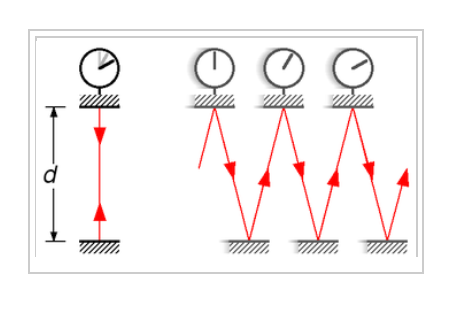This light clock is associated with

A

special relativity

B

gravitational shift

C

doppler shift

D

general relativity

E

all of these are true

Q30

The range of wavelength for visible light is between

A

600 and 1200 nanometers

B

1 and 10 nanometers

C

5000 and 6000 nanometers

D

400 and 700 nanometers

E

0.1 and 10 nanometers

Q31

What causes the blue glow of the Crab nebula?

A

the Doppler blue shift

B

the curving motion of electrons in a magnetic field; such motion resembles a radio antenna

C

the Gravitational blue shift

D

the same emission found in a Lava lamp (ultraviolet)

E

the curving motion of electrons in a magnetic field; such motion traps ultraviolet and blue light

Q32

The course materials present two cosmic expansion plots. Hubble's original (1929) plot used

A

supernovae

B

novae

C

red giants

D

Cepheid variables

E

entire galaxies

Q33

Which of the following changes in the properties of a giant molecular cloud might cause it to collapse?

A

Decrease mass at fixed temperature and size

B

Two of these are correct

C

Increase mass at fixed temperature and size

D

Increase size at fixed pressure and mass

E

Increase temperature at fixed mass and size

Q34

Many stars in a typical globular cluster are nearly as old as the universe

A

True

B

False

Q35

Why is a star made of plasma?

A

it is so hot that electrons are stripped away from the protons

B

the interstellar gas was mostly plasma

C

plasma is generic word for "important"

D

plasma is always present when there are strong magnetic fields

E

the intense gravity liquifies the substance, just as red blood cells liquify plasma in the body